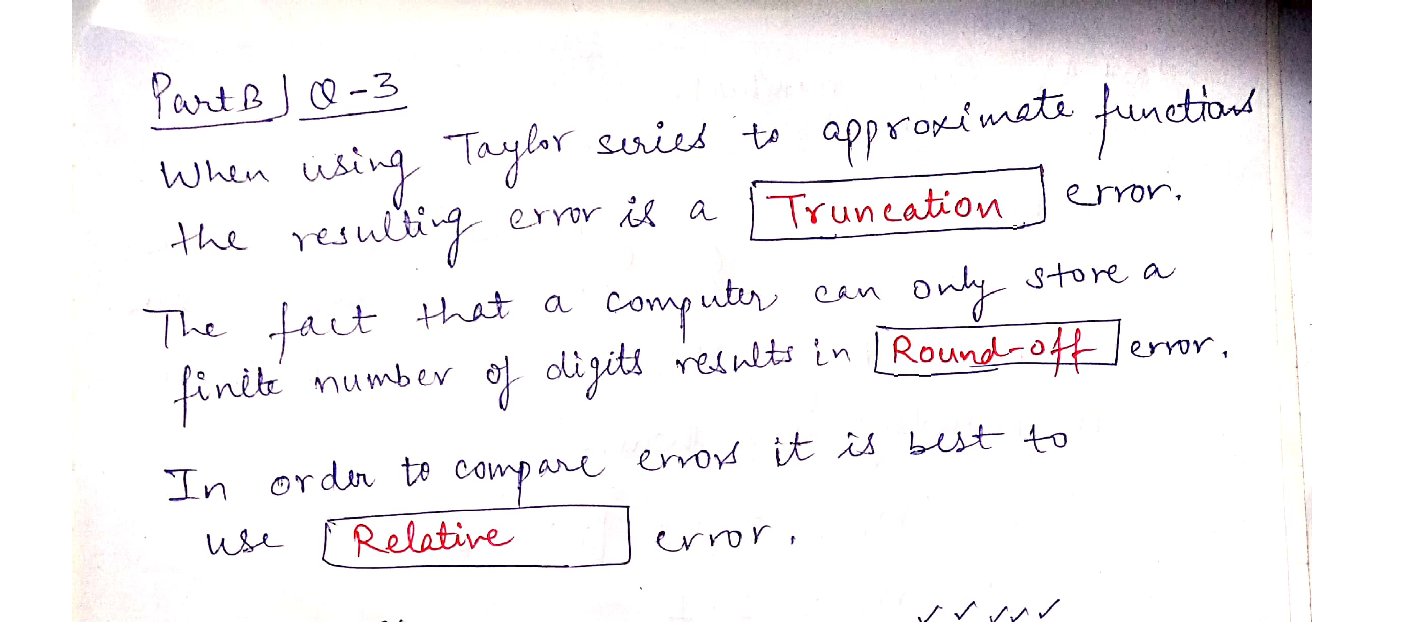Question

Numerical Methods

When using Taylor series to approximate functions, the resulting error is error

The fact that a computer can only store a finite number of digits results in errors

In order to compare errors it is best to use errors.Verified### Question 38315Numerical Methods

For a long, thin insulated rod, a parabolic equation can be employed to characterize time -variable problems for a heat conduction equation in one dimensional which requires approximation for the second derivative in space and the first derivative in time.
а.In the calculation of heat conduction equation, describe the assumption that need to be considered.
b.For one dimensional heat equation in insulated thin rod, derive equations for the first, internal and the last point of the grid.
d.Select thebest method from the TWO (2) numerical methods in Part (2)(c). Justify your answer.

### Question 38314Numerical Methods

In the process of making a mixture, the concentration of sodium x g/L is given as a function of time by
\frac{d x}{d t}=28-2.5 x
Given the initial time, t = 0 minute, the concentration of sodium in the tank is 45| L. Choose a step size in a range of 1 <h< 3 minutes for the interval from 0 to 3h minutes,
а.Calculate the sodium concentration using the TWO (2) numerical methods known as Runge Kutta 4th order method and Euler's method.
b.Describe ONE (1) advantage and ONE (1) disadvantage for the TWO(2) numerical methods in Part (1)(a) respectively.
c.Determine the best method from the TWO (2) numerical methods in Part (1)(b) based on true percent relative error. Justify your answer.

### Question 35737Numerical Methods

. The differential equation describing heat conduction,
\frac{\partial u}{\partial t}=c^{2} \frac{\partial^{2} u}{\partial x^{2}}, \quad 0 \leq x \leq 1, \quad t \geq 0
subject to the conditions
\frac{\partial u}{\partial x}(0, t)=0, \quad u(1, t)=9 \cos (1) e^{-c^{2} t}, \quad t \geq 0
and
u(x, t)=9 e^{-c^{2} t} \cos x
has the (exact) solution
u(x, 0)=9 \cos x, \quad 0 \leq x \leq 1

### Question 33307Numerical Methods

In this example of the free-falling parachutist, we assumed that the acceleration due to gravity was a constant value. Although this is a decent approximation when we are examining falling objects near the surface of the earth, the gravitational force decreases as we move above sea level. A more general representation based on Newton's inverse square law of gravitational attraction can be written as presented in Eq.1
g(x)=g(0) \frac{R^{2}}{(R+x)^{2}}
\frac{d v}{d t}=g-\frac{c}{m} v
where g(x) = gravitational acceleration altitude x (in m) measured upward from the earth's surface (m/s²), g(0)=gravitational acceleration at the earth's surface (9.81 m/s³),and R = the earth’s radius (6.37 x 106 m).
(a)In a fashion similar to the derivation of (Eq. 2) use a force balance to derive a differential equation for velocity as a function of time that utilizes this more complete representation of gravitation. However, for this derivation, assume that upward velocity is positive. (20 points)
(b)For the case where drag is negligible, use the chain rule to express the differential equation as a function of altitude rather than time. Recall that the chain rule is:
\frac{d v}{d t}=\frac{d v}{d x} \frac{d x}{d t} \text { (25 points) }
(c)Use calculus to obtain the closed form solution where v = vo at x = 0.
(d)Use Euler's method to obtain a numerical solution from x = 0 to 100.000 m.using a step of 10,000 m where the initial velocity is 1500 m/s upward. (25
(e)Compare your result with the analytical solution.

### Question 30331Numerical Methods

1. (20 pts.) (Problem 1.5): Compute the velocity of a free-falling parachutist using Euler's method for the case where m= 80kg and c = 10kg/s. Derive the mathematical model and perform the calculation from t = 0 to 20 s with a step size of 1 s. Use an initial condition that the parachutist has an upward velocity of 20 m/s at t = 0. At t = 10 s, assume that the chute is instantaneously deployed so that the drag coefficient jumps to 50 kg/s. (Show all steps)

### Question 25904Numerical Methods

When estimating Euler's number e using this Taylor series, the truncation error is (use the value provided by your hand calculator or octave/Matlab as a reference for Euler's number e)
Expand the exponential function e around x = 0 in a Taylor series of order 4.
a. 0.0099485
b. 2.7183
C. -0.051615
d. 0.051615
e. -0.0099485

### Question 25903Numerical Methods

Numerical algorithms provide approximations of mathematical problems.
d. They estimate the total error (sum of truncation and round-off errors)
b. They estimate truncation errors
a. They estimate round-off errors
c. They don't estimate errors
They estimate the following type of errors:

### Question 25902Numerical Methods

How many significant figures are in the number 2.5000?

### Question 25901Numerical Methods

When using Taylor series to approximate functions, the resulting error is error
The fact that a computer can only store a finite number of digits results in errors
In order to compare errors it is best to use errors.

### Question 25900Numerical Methods

When adding the three numbers 2.12, 0.8 and 1.033 (all reported with maximal significant figures) how many significant figures will have the answer?

### Submit query

Getting answers to your urgent problems is simple. Submit your query in the given box and get answers Instantly.

### Submit a new QuerySuccess

Assignment is successfully created